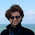## Monday, January 07, 2013

### SODA 2013: Part 3/n

I just attended Valerie King's talk on her paper with Bruce Kapron and Ben Mountjoy that does dynamic connectivity in worst-case polylog time (randomized). This paper received a Best Paper award (along with the Grohe et al paper on graph minors I mentioned yesterday).

The problem is a classic: given a stream of updates (edge insertions/deletions) to a graph, maintain a data structure that can answer "Are u and v connected" efficiently. There's been a slew of work on the problem: if you want worst-case bounds for updates, the best till now was $O(\sqrt{n})$ by Eppstein, Galil, Italiano and Nissenzweig (from 1992). There are polylogarithmic amortized bounds, but they can incur worst-case update times of $\Theta(n)$.

In this paper, after 20 years, they knock down the worst-case update times to polylogarithmic: the algorithms are randomized (with 1-sided error: it might occasionally declare two nodes disconnected when they were connected).

The idea itself is very elegant, and it connects to techniques in streaming algorithms in a way that I think is neat. WARNING: IANAE in this area, so my rendition might be slightly naïve, and is drawn from my understanding of Valerie King's excellent presentation.

The basic problem is this: I can maintain connectivity by maintaining a collection of trees. Adding an edge can be done without too much difficulty (indeed, if all you want to do is insert things, then you're back to union-find). It's insertion and deletion together that makes things hard: imagine that you delete an edge and disconnect a tree: you need to quickly determine if there's some other non-tree edge that can be used to reconnect the pieces, or if the two sides are now really disconnected.

More generally, consider the cutset maintainence problem. You have updates as before, but now a query is: given a set S, find a cut edge if one exists between S and V - S. It's not hard to see that this is the core routine you need for the tree.

The first elegant idea is this: suppose you represent each edge by a bit string consisting of the encoding of one endpoint followed by an encoding of the other. For example, the edge 2-3 might be written as 010011. Now for each vertex, compute the XOR of all the edges adjacent to it and call this its signature.

If you XOR all the signatures of vertices in a set, and if the set has exactly one cut edge, then what you get is the signature of that edge ! This is because each edge that's inside S will occur twice and therefore will zero out.

So this works if your cut set is of size 1. But suppose it's not ? Now you maintain $O(\log n)$ sets for each vertex. Each edge adjacent to the vertex is thrown into the $i^{\text{th}}$ set with probability $1/2^i$. With some appropriate duplication, you can show that if the cut set is of size $k$, then w.h.p the $\log k$th cut set will have exactly one element in it, and that's the element you can retrieve when you need to find a replacement edge.

There's a lot more technical work to do to get the whole algorithm working, and I won't go into that. But those of you who are familiar with streaming will recognize something here.

In essence, they've created a sketch for the set of edges adjacent to the vertex, and they have a way to represent the set compactly and retrieve individual elements from it. The trick with exponentially decaying levels is standard in streaming count estimation, and the GF(2) trick for storing the sketch is very similar to the trick used by Ahn, Guha and McGregor in their SODA 2012 paper on graph sketching.

And that's what I thought was the best part: that ideas that really have power in streaming can be used to help solve a longstanding open problem in graph algorithms. I should point out that the connection is post-facto: this paper was developed independently of the streaming work. But one does have to wonder what other connections we might be able to establish between sketching tricks on graphs and basic graph algorithms.

1.Do you know if any analogous results are known for dynamic directed graph reachability?

2.There's a STOC 2004 paper by Roddity and Zwick and a FOCS 2004 paper by Piotr Sankowski. But the bounds there are weaker: no polylog updates.

3.There are good reasons to believe that it may be impossible to do dynamic graph reachibility with poly-logarithmic update/query time:
http://people.csail.mit.edu/mip/papers/mp-3sum/paper.pdf